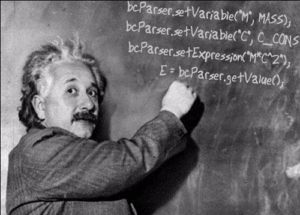# Math Expression Parsers

Computer programs easily compute mathematical expressions that they contain. Some interpreted languages provide an eval() function that you may use however insecure it may be. But often we the programmers feel the need to evaluate math formulas given as strings at runtime. Some of our domain specific applications do need to allow the users enter formulas to be parsed and evaluated at runtime.We have formula parsers for many programming languages

Few examples are engineering applications that require the users not only enter parameter values for pre-defined formulas, but change those formulas themselves in the first place. For example a mechanical engineering CAD/CAM design application could allow the user enter stress, strain, temperature but also allow the user to sepcify the relationships between these values in the form of a math expression. A simplex method implementation that solves an equation could allow the user input the equations interactively. A database engine implementation by nature has it’s own parser, so do the various interpreted languages such as PHP, Ruby, Python, JavaScript.
Same can’t be told for compiled languages though. Ruby solution would still require alot of plumbing code to get it secure even though there is a eval function in Ruby. Same thing can be told for PHP where a website user could enter any PHP expression as a formula and if you were to take it as is, you would expose your website to hackers if you executed the math formula as is.
There are other challenges of parsing the formula in compiled languages. Compiled languages such as C, C++, C#, VB, Delphi, Pascal, Java do not have a built in way to evaluate expressions at runtime in the context of the application. Thus if you need such capability, you need to build it yourself. The work requires parsing a user defined string that is a math formula into it’s sub-components such as variables, constants, operators, function calls and so on and then evaluate it to produce an answer based on user defined values and implementations for these variables and functions.
Luckily, we have ready to use, tested and proven math expression parser components for all of these languages. It is usually better to re-use code than invent it yourself. Pick your choice on the right. We have it for many languages.

## Math Parser LibrariesTbcParser is a Delphi component that can be used with Delphi and Borland C++ Builder. (Source code is included.)JbcParser is math expression parser library for Java. Works with Java 5 and above. (Source code is included.)bcParser.NET is the math parser component for .NET. It can be used with all .NET languages including VB.NET and C# (C# Source code is included)bcParserGo is the formula parser for Golang. Comes as Go source code that can compile on Windows, Linux, Mac.bcParserCPP is the formula parser for C++. Comes as MathParser.h C++ template header file. Good for C++ projects with Visual C++, C++ Builder, GNU C++, Xcode on Windows, Linux, Mac.bcParserObjC is the formula parser for Objective C. Comes as MathParser.h, MathParser.m source files. Good for Objective C projects for iPhone, iPad and Mac OSX.bcParserPHP is the formula parser for PHP. Comes as MathParser.php source code, it can be used in your PHP applications in a safe way because it does not have the security holes of eval().
Why re-invent the wheel? Buy our components at very affordable prices and save valuable time.